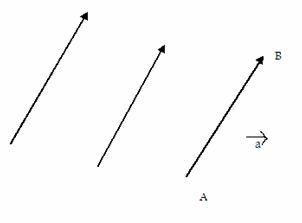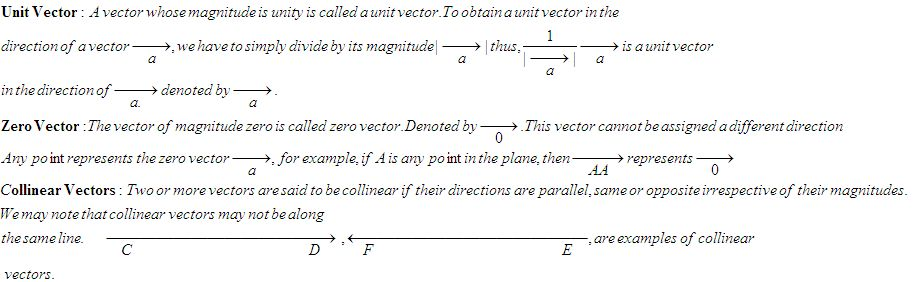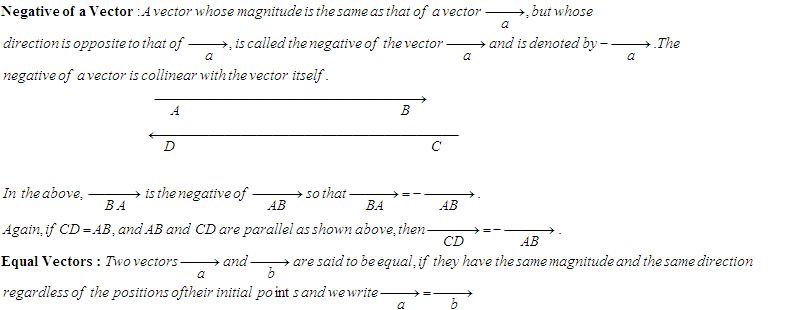Name: ___________________Date:___________________

 Email us to get an instant 20% discount on highly effective K-12 Math & English kwizNET Programs!

### High School Mathematics - 25.4 Vectors

 Vectors can be used to represent quantities that involve both direction and magnitude. There are also quantities whic have magnitude but not direction. These quantities are called scalars.In the above figure we have three parallel straight lines and there are two different directions that can be associated with these lines as shown by arrowheads. A line with one of these directions prescribed is called a directed line. If a magnitude , say a units in the form of line segment on this line together with one of the two directions on the line are prescribed, we say that we have a directed line segment . In the third line, AB, A is called the initial point and B is called the terminal point of the directed line segment.Since the directed line segment has both magnitude and direction, we call it a vector. Magnitude of a vector is written as |->| a We employ the symbol AB with a ray symbol above it or a with a ray symbol above to denote the vector. Types of vectorsExample: Find the magnitude of | 3i + 2 j +4 k| Solution: Ö(32+22+42 Answer: Magnitude is Ö17 Directions: Answer the following.Name: ___________________Date:___________________

### High School Mathematics - 25.4 Vectors

 Q 1: Vectors having the same magnitude and direction are called ____ vectors.unitequalzero Q 2: A negative vector has the same direction as that of the given vector but different magnitude.FalseTrue Q 3: Compute |i+j+k| 35Ö3 Q 4: Vectors are physical quantities that have _____magnitudeboth magnitude and directiondirection Q 5: Scalars are physical quantities that havedirectionbothmagnitude Q 6: Acceleration is a ____ quantity.scalarvector Q 7: Speed is ____ quantity.vectorscalar Q 8: Which of these is a vector quantity?VelocityTemperatureMassTime Question 9: This question is available to subscribers only! Question 10: This question is available to subscribers only!